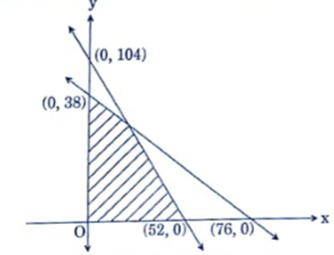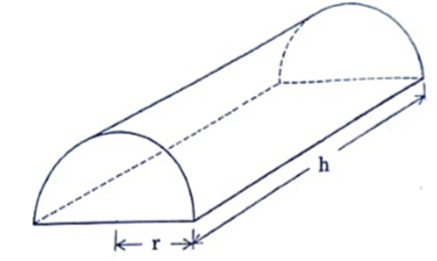Request a call back

# CBSE Class 12 Mathematics Previous Year Question Paper Term 1 2021 SSJ-1-Set-4

Your CBSE Class 12 syllabus for Maths consists of topics such as linear programming, vector quantities, determinants, etc. which lay the foundation for further education in science, engineering, management, etc. Studying differential equations will be useful for exploring subjects such as Physics, Biology, Chemistry, etc. where your knowledge can be applied for scientific investigations.

On TopperLearning, you can find study resources such as sample papers, mock tests, Class 12 Maths NCERT solutions and more. These learning materials can help you understand concepts such as differentiation of functions, direction cosines, integrals, and more. Also, you can practise the Maths problems by going through the solutions given by our experts.

Maths is considered as one of the most difficult subjects in CBSE Class 12 Science. Our Maths experts simplify complex Maths problems by assisting you with the right methods to solve problems and score full marks. You may still have doubts while referring to the Maths revision notes or Maths NCERT solutions. Solve those doubts by asking an expert through the “Undoubt” feature on the student dashboard.

SECTION A

In this section, attempt any 16 questions out of questions no. 1 to 20.

Question 1.

A relation R is defined on N. Which of the following is the reflexive relation?

1. R = {(x, y): x > y; x, y ∊ N}
2. R = {(x, y): x + y = 10; x, y ∊ N)}
3. R = {(x, y): xy is the square number; x, y ∊ N}
4. R = {(x, y): x + 4y = 10; x, y ∊ N}

Question 2.

The function f: R ⟶ R defined by f(x) = 4 + 3 cos x is:

1. bijective
2. one-one but not onto
3. onto but not one-one
4. neither one-one nor onto

Question 3.

If y = cot-1 x, x < 0, then:

1.2.3.4.Question 4.

The number of functions defined from {1, 2, 3, 4, 5} ⟶ {a, b} which are one-one is:

1. 5
2. 3
3. 2
4. 0

Question 5.

If A =, then (A – 2I) (A – 3I) is equal to:

1. A
2. I
3. 5I
4. O

Question 6.

If P is a 3 x 3 matrix such that P’ = 2P + I, where P’ is the transpose of P, then:

1. P = I
2. P = –I
3. P = 2I
4. P = –2I

Question 7.

If order of matrix A is 2 x 3, of matrix B is 3 x 2, and of matrix C is 3 x 3, then which one of the following is not defined?

1. C (A + B’)
2. C (A + B’)’
3. BAC
4. CB + A'

Question 8.

If A =and |A3| = 27, then the value of α is:

1.2.3.4.Question 9.= 0, then the value of x is:

1. 3
2. 5
3. 7
4. 9

Question 10.

The inverse ofis:

1.2.3.4.Question 11., then A–1:

1. is A
2. is (-A)
3. is A2
4. does not exist

Question 12.

If the functionis continuous, then the value of k is:

1.2.3.4.Question 13.

The function f(x) = [x], where lx] is the greatest integer function that is less than or equal to x, is continuous at:

1. 4
2. –2
3. 1.5
4. 1

Question 14.

If y = tan–1(e2x), thenis equal to:

1.2.3.4.Question 15.

If y2 (2 – x) = x3,  thenis equal to:

1. 2
2. –2
3. 3
4.Question 16.

The angle between the tangents to the curve y = x2 – 5x + 6 at the points (2, 0) and (3, 0) is:

1.2.3.4. 0

Question 17.

The interval, in which function y = x3 + 6x2 + 6 is increasing is:

1. (–∞, –4) U (0, ∞)
2. (–∞, 4)
3. (–4, 0)
4. (–∞, 0) U (4, ∞)

Question 18.

The value of x for which (x – x2) is maximum, is:

1.2.3.4.Question 19.

If the corner points of the feasible region of an LPP are (0, 3), (3, 2) and (0, 5), then the minimum value of Z = 11x + 7y is:

1. 21
2. 33
3. 14
4. 35

Question 20.

The number of solutions of the system of inequations x + 2y ≤ 3, 3x + 4y ≥ 12, x ≥ 0, y ≥ 1 is:

1. 0
2. 2
3. finite
4. infinite

SECTION B

In this section, attempt any 16 questions out of questions no. 21 to 40.

Question 21.

The number of equivalence relations in the set {1, 2, 3} containing the elements (1, 2) and (2, 1) is:

1. 0
2. 1
3. 2
4. 3

Question 22.

Let f: R ⟶ R be defined by f(x) =, for all x ∊ R. Then, f is:

1. one-one
2. onto
3. bijective
4. not defined

Question 23.

The function f: N ⟶ N is defined by f(n) =The function f is:

1. bijective
2. one-one but not onto
3. onto but not one-one
4. neither one-one nor onto

Question 24.

The value of sin–1is:

1.2.3.4.Question 25.

If sin–1 x > cos–1 x, then x should lie in the interval:

1.2.3.4.Question 26.

If A =and A + A’ = I, then the value of α is:

1.2.3.4.Question 27.

The determinantis equal to:

1. k(3y + k2)
2. 3y + k3
3. 3y + k2
4. k2(3y + k)

Question 28.

If A =is the adjoint of a square matrix B, then B-1 is equal to:

1. ±A
2. ±√2A
3.4.Question 29.

If A =, then A5 – A4 – A3+ A2 is equal to

1. 2A
2. 2A
3. 4A
4. O

Question 30.

If y = e-x, thenis equal to:

1. –y
2. y
3. x
4. –x

Question 31.

If x = t2 + 1, y = 2at, thenat t = a is:

1.2.3.4. 0

Question 32.

The functionis:

1. not differentiable at x = 1
2. differentiable at x = 1
3. not continuous at x = 1
4. neither continuous nor differentiable at x = 1

Question 33.

The curve x2 – xy + y2 = 27 has tangents parallel to x-axis at:

1. (3, 6) and (–3, –6)
2. (3, –6) and (–3, 6)
3. (–3, –6) and (3, –6)
4. (–3, 6) and (–3, –6)

Question 34.

A wire of length 20 cm is bent in the form of a sector of a circle. The maximum area that can be enclosed by the wire is:

1. 20 sq cm
2. 25 sq cm
3. 10 sq cm
4. 30 sq cm

Question 35.

The function (x – sin x) decreases for:

1. all x
2.3.4. no value of x

Question 36.

If θ is the angle of intersection between the curves y2 = 4ax and ay = 2x2 at (a, 2a),  then the value of tan θ is:

1.2.3.4.Question 37.

The maximum value of Z = 3x + 4y subject to the constraints x ≥ 0, y ≥ 0 and x + y ≤ 1 is:

1. 7
2. 4
3. 3
4. 10

Question 38.

The feasible region of an LPP is given in the following figure:Then, the constraints of the LPP are x ≥ 0, y ≥ 0 and

1. 2x + y ≤ 52 and x + 2y ≤ 76
2. 2x + y ≤ 104 and x + 2y ≤ 76
3. x + 2y ≤ 104 and 2x + y ≤ 76
4. x + 2y ≤ 104 and 2x + y ≤ 38

Question 39.

If the minimum value of an objective function Z =  ax + by occurs at two points (3, 4) and (4, 3), then:

1. a + b = 0
2. a = b
3. 3a = b
4. a = 3b

Question 40.

For the following LPP
Maximise Z = 3x + 4y
Subject to constraints
x – y ≥ -1, x ≤ 3
x ≥ 0, y ≥ 0
The maximum value is:

1. 0
2. 4
3. 25
4. 30

SECTION C

In this section, attempt any 8 questions out of questions no. 41 – 50.8 × 1 = 8

Question 41.

A relation R is defined on Z as:
aRb if and only if a2 – 7ab + 6b2 = 0.
Then, R is:

1. reflexive and symmetric
2. symmetric but not reflexive
3. transitive but not reflexive
4. reflexive but not symmetric

Question 42.

The value ofis:

1. 12
2. –12
3. 24
4. –24

Question 43.1. a = 1 = b
2. a = cos 2θ, b = sin 2θ
3. a = sin 2θ, b = cos 2θ
4. a = cos θ, b = sin θ

Question 44.

The normal to the curve 3y = 6x – 5x3 at the  pointpasses through the point:

1. (3, 1)
2. (3, 2)
3. (2, 3)
4. (1, 1)

Question 45.

If y = sin(2 sin-1x), then (1 – x2)y2 is equal to:

1. –xy1 + 4y
2. –xy1 – 4y
3. xy1 – 4y
4. xy1 + 4y

Case Study

Some young entrepreneurs started an industry “young achievers” for casting metal into various shapes. They put up an advertisement online stating the same and expecting order to cast metal for toys sculptures, decorative pieces and more.
A group of friends wanted to make innovative toys and hence contacted the “young achievers” to order them to cast metal into solid half cylinders with a rectangular base and semi-circular ends.Based on the above information, answer the following questions:

Question 46.

The volume (V) of the casted half cylinder will be:

1. πr2h
2.3.4.  πr2(r + h)

Question 47.

The total surface area (S) of the casted half cylinder will be:

1. πrh + 2πr2 + rh
2. πrh + πr2 + 2rh
3. 2πrh + πr2 + 2rh
4. πrh + πr2 + rh

Question 48.

The total surface area S can be expressed in terms of V and r as:

1.2.3.4.Question 49.

For the given half-cylinder of volume V, the total surface area S is minimum, when:

1. (π + 2)V = π2r3
2. (π + 2)V = π2r2
3. 2(π + 2)V = π2r3
4. (π + 2)V =π2r

Question 50.

The ratio h : 2r for S to be minimum will be equal to:

1. 2π : π + 2
2. 2π : π + 1
3. π : π + 1
4. π : π + 2

## Why CBSE Class 12 Science Maths solutions are important?

Maths is a subject which requires practising a variety of problems to understand concepts clearly. By solving as many problems as you can, you’ll be able to train your brain in thinking the logical way to solve maths problems. For practising problems, study materials such as sample papers, previous year papers, and NCERT solutions are needed.

Some of the best Maths experts work with us to give you the best solutions for Maths textbook questions and sample paper questions. Chapter-wise NCERT solutions for Class 12 Science Maths can be easily accessible on TopperLearning. Use these solutions to practise problems based on concepts such as direction ratios, probability, area between lines, inverse trigonometric functions, and more.

To prepare for your Maths exam, you need to attempt solving different kinds of Maths questions. One of the best ways to assess your problem-solving abilities is to attempt solving previous year papers with a set timer. Our Maths solutions will come in handy to help you with checking your answers and thus, improving your learning experience. So, to score more marks in your Class 12 board exams, use our Maths solutions that will enable you with the appropriate preparation.